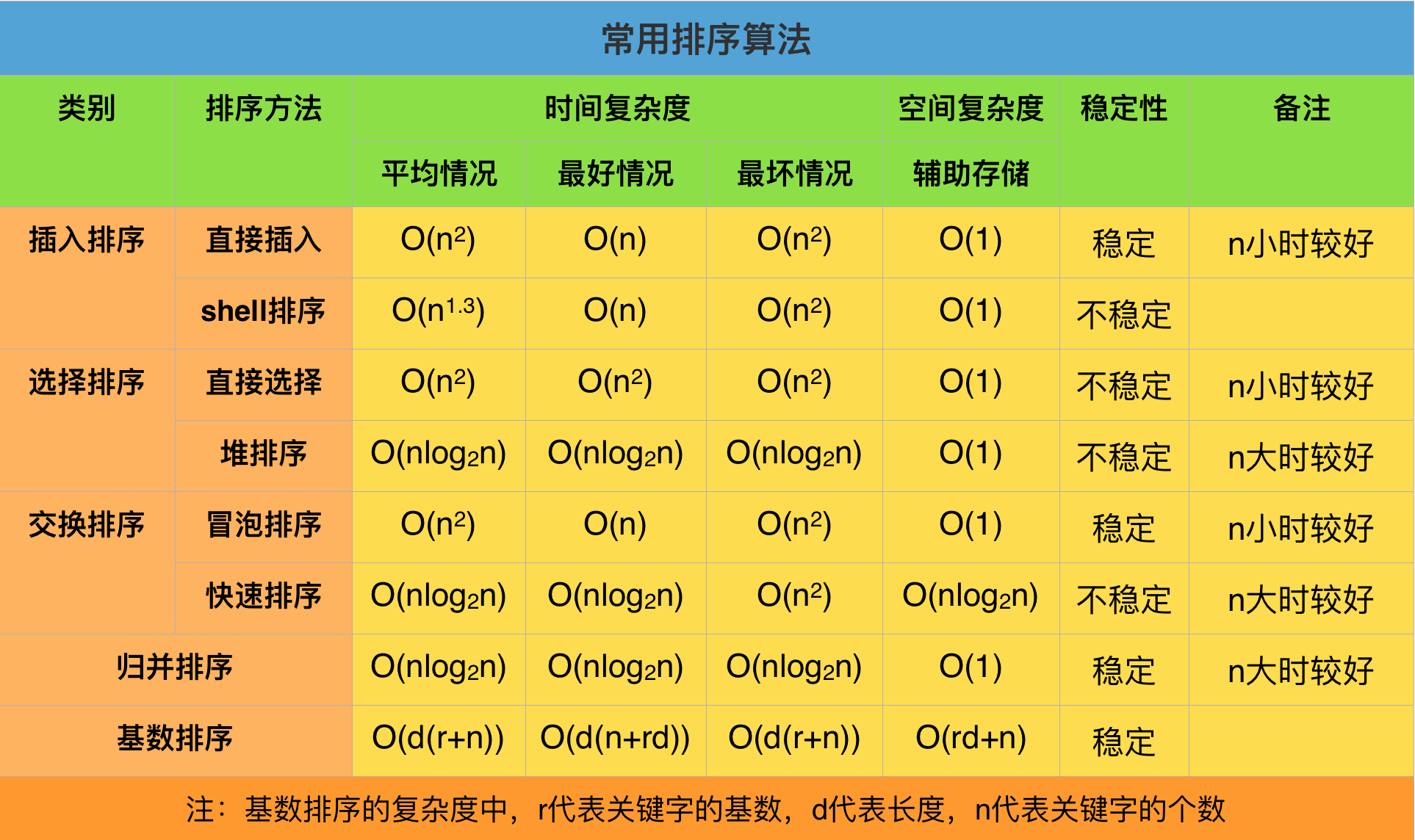八大排序算法总结与java实现

概述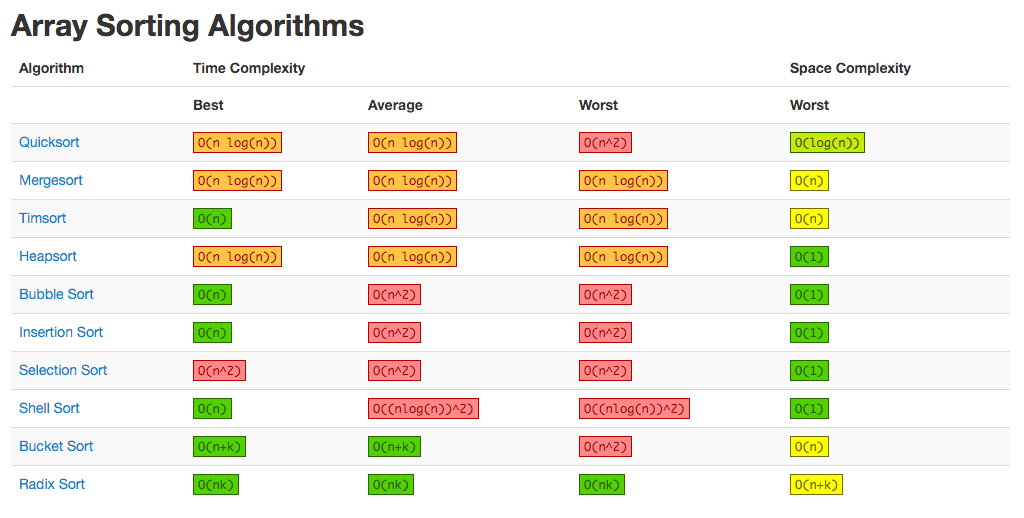• 直接插入排序
• 希尔排序
• 简单选择排序
• 堆排序
• 冒泡排序
• 快速排序
• 归并排序
• 基数排序

$$\begin{cases} 内部排序 \begin{cases} 插入排序 \begin{cases} 直接插入排序\\ 希尔排序 \end{cases} \\ 选择排序 \begin{cases} 简单选择排序\\ 堆排序 \end{cases} \\ 交换排序 \begin{cases} 冒泡排序\\ 快速排序 \end{cases} \\ 归并排序\\ 基数排序 \end{cases} \\ 外部排序 \end{cases}$$

一、直接插入排序（Insertion Sort）

1、基本思想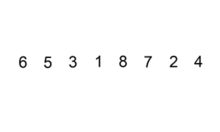2、算法描述

①. 从第一个元素开始，该元素可以认为已经被排序
②. 取出下一个元素，在已经排序的元素序列中从后向前扫描
③. 如果该元素（已排序）大于新元素，将该元素移到下一位置
④. 重复步骤3，直到找到已排序的元素小于或者等于新元素的位置
⑤. 将新元素插入到该位置后
⑥. 重复步骤②~⑤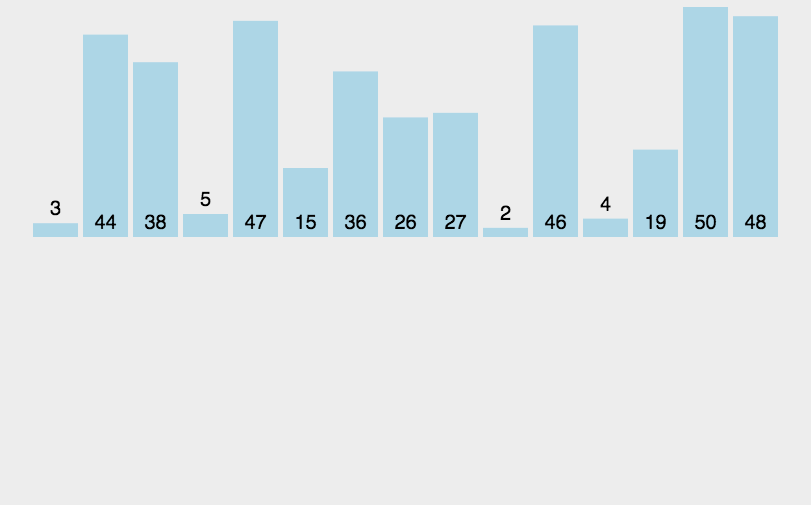3、代码实现

• 最好情况下，排序前对象已经按照要求的有序。比较次数(KCN)：$n−1$；移动次数(RMN)为$0$。则对应的时间复杂度为$O(n)$。
• 最坏情况下，排序前对象为要求的顺序的反序。第i趟时第i个对象必须与前面i个对象都做排序码比较，并且每做1次比较就要做1次数据移动（从上面给出的代码中看出）。比较次数(KCN)：$\sum_{i=1}^{n-1}i=\frac{n(n-1)}{2}\approx \frac{n^{2}}{2}$ ; 移动次数(RMN)为：$\sum_{i=1}^{n-1}i=\frac{n(n-1)}{2}\approx \frac{n^{2}}{2}$。则对应的时间复杂度为$O(n^{2})$。
• 如果排序记录是随机的，那么根据概率相同的原则，在平均情况下的排序码比较次数和对象移动次数约为$\frac{n^{2}}{2}$，因此，直接插入排序的平均时间复杂度为$O(n^{2})$。

O(n²) O(n) O(n²) O(1)

Tips: 由于直接插入排序每次只移动一个元素的位， 并不会改变值相同的元素之间的排序， 因此它是一种稳定排序。

二、希尔排序（Shell Sort）

1、基本思想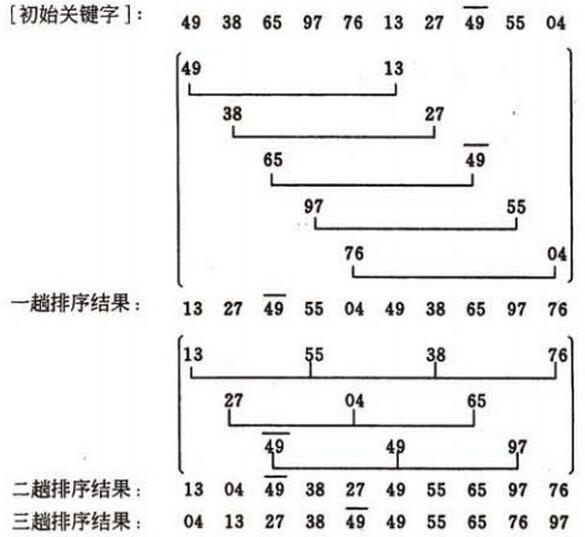2、算法描述

①. 选择一个增量序列t1，t2，…，tk，其中ti>tj，tk=1；（一般初次取数组半长，之后每次再减半，直到增量为1
②. 按增量序列个数k，对序列进行k 趟排序；
③. 每趟排序，根据对应的增量ti，将待排序列分割成若干长度为m 的子序列，分别对各子表进行直接插入排序。仅增量因子为1 时，整个序列作为一个表来处理，表长度即为整个序列的长度。

3、代码实现

①. 第一层for循环表示一共有多少个增量。增量的序列的个数，就是希尔排序的趟数。上面的增量序列为： arr.length/2, arr.length/2/2, arr.length/2/2/2, .... 2, 1
②. 里层的两个for循环，实际上就是以一个gap拆分为一组的组内插入排序

O(nlog2 n) O(nlog2 n) O(nlog2 n) O(1)

三、选择排序（Selection Sort）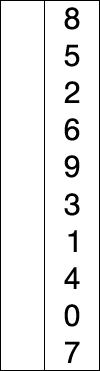2、算法描述

①. 从待排序序列中，找到关键字最小的元素；
②. 如果最小元素不是待排序序列的第一个元素，将其和第一个元素互换；
③. 从余下的 N - 1 个元素中，找出关键字最小的元素，重复①、②步，直到排序结束。

3、代码实现

O(n²) O(n²) O(n²) O(1)

四、堆排序（Heap Sort）

1991年的计算机先驱奖获得者、斯坦福大学计算机科学系教授罗伯特·弗洛伊德(Robert W．Floyd) 和威廉姆斯(J．Williams) 在1964年共同发明了著名的堆排序算法(Heap Sort).

\left\{ \begin{aligned} k_i & \leqslant k_{2i} \\ k_i & \leqslant k_{2i+1} \end{aligned} \right. \quad 或 \quad \left\{ \begin{aligned} k_i & \geqslant k_{2i} \\ k_i & \geqslant k_{2i+1} \end{aligned} \right. \quad (i = 1,2,···, \left \lfloor { \frac{n}{2} } \right \rfloor )

2、算法描述

①. 先将初始序列$K[1..n]$建成一个大顶堆, 那么此时第一个元素$K_1$最大, 此堆为初始的无序区.
②. 再将关键字最大的记录$K_1$ (即堆顶, 第一个元素)和无序区的最后一个记录 $K_n$ 交换, 由此得到新的无序区$K[1..n-1]$和有序区$K[n]$, 且满足$K[1..n-1].keys \leqslant K[n].key$
③. 交换$K_1$ 和 $K_n$ 后, 堆顶可能违反堆性质, 因此需将$K[1..n-1]$调整为堆. 然后重复步骤②, 直到无序区只有一个元素时停止.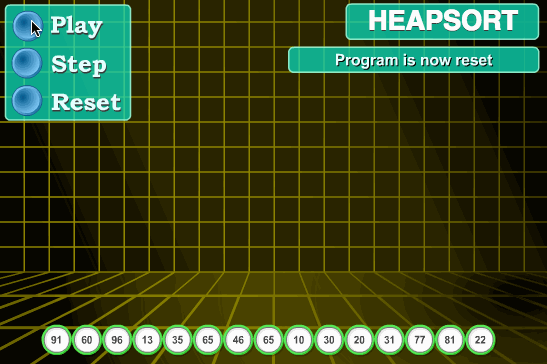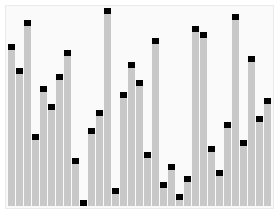3、代码实现

• 最大堆调整（Max_Heapify）：将堆的末端子节点作调整，使得子节点永远小于父节点
• 创建最大堆（Build_Max_Heap）：将堆所有数据重新排序
• 堆排序（HeapSort）：移除位在第一个数据的根节点，并做最大堆调整的递归运算

• 父节点i的左子节点在位置：(2*i+1);
• 父节点i的右子节点在位置：(2*i+2);
• 子节点i的父节点在位置：floor((i-1)/2);

①. 建立堆的过程, 从length/2 一直处理到0, 时间复杂度为O(n);
②. 调整堆的过程是沿着堆的父子节点进行调整, 执行次数为堆的深度, 时间复杂度为O(lgn);
③. 堆排序的过程由n次第②步完成, 时间复杂度为O(nlgn).

$O(n \log_{2}n)$ $O(n \log_{2}n)$ $O(n \log_{2}n)$ O(1)

Tips: 由于堆排序中初始化堆的过程比较次数较多, 因此它不太适用于小序列. 同时由于多次任意下标相互交换位置, 相同元素之间原本相对的顺序被破坏了, 因此, 它是不稳定的排序.

五、冒泡排序（Bubble Sort）1、基本思想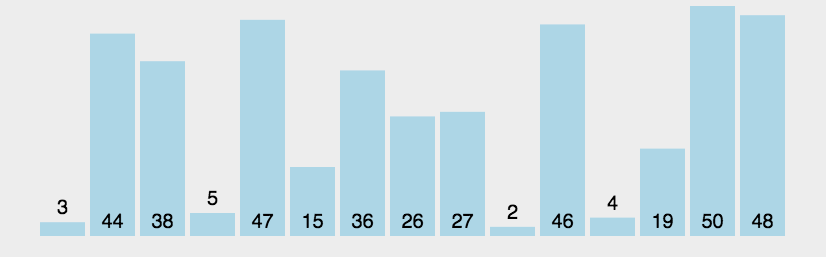2、算法描述

①. 比较相邻的元素。如果第一个比第二个大，就交换他们两个。
②. 对每一对相邻元素作同样的工作，从开始第一对到结尾的最后一对。这步做完后，最后的元素会是最大的数。
③. 针对所有的元素重复以上的步骤，除了最后一个。
④. 持续每次对越来越少的元素重复上面的步骤①~③，直到没有任何一对数字需要比较。

3、代码实现

O(n²) O(n) O(n²) O(1)

Tips: 由于冒泡排序只在相邻元素大小不符合要求时才调换他们的位置, 它并不改变相同元素之间的相对顺序, 因此它是稳定的排序算法.

六、快速排序（Quick Sort）

1、基本思想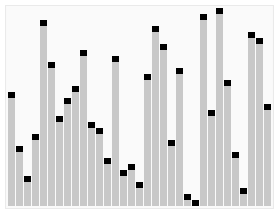2、算法描述

①. 从数列中挑出一个元素，称为”基准”（pivot）。
②. 重新排序数列，所有比基准值小的元素摆放在基准前面，所有比基准值大的元素摆在基准后面（相同的数可以到任一边）。在这个分区结束之后，该基准就处于数列的中间位置。这个称为分区（partition）操作。
③. 递归地（recursively）把小于基准值元素的子数列和大于基准值元素的子数列排序。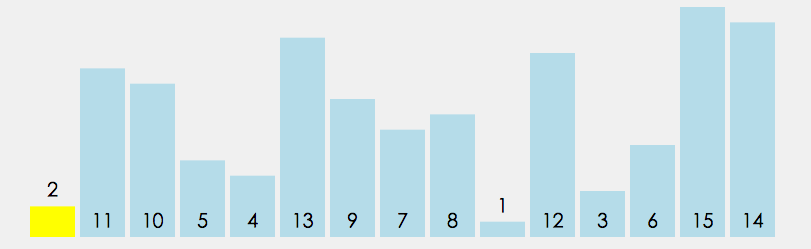3、代码实现

①. i = L; j = R; 将基准数挖出形成第一个坑a[i]
②．j--，由后向前找比它小的数，找到后挖出此数填前一个坑a[i]中。
③．i++，由前向后找比它大的数，找到后也挖出此数填到前一个坑a[j]中。
④．再重复执行②，③二步，直到i==j，将基准数填入a[i]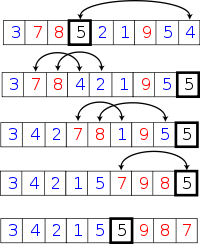O(nlog₂n) O(nlog₂n) O(n²) O(1)（原地分区递归版）

Tips: 同选择排序相似, 快速排序每次交换的元素都有可能不是相邻的, 因此它有可能打破原来值为相同的元素之间的顺序. 因此, 快速排序并不稳定.

七、归并排序（Merging Sort）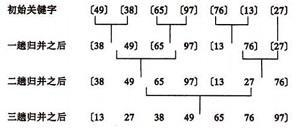1、基本思想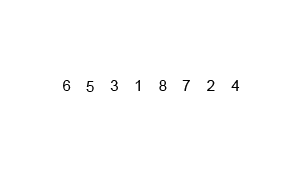2、算法描述

• 自上而下的递归
• 自下而上的迭代

①. 将序列每相邻两个数字进行归并操作，形成 floor(n/2)个序列，排序后每个序列包含两个元素；
②. 将上述序列再次归并，形成 floor(n/4)个序列，每个序列包含四个元素；
③. 重复步骤②，直到所有元素排序完毕。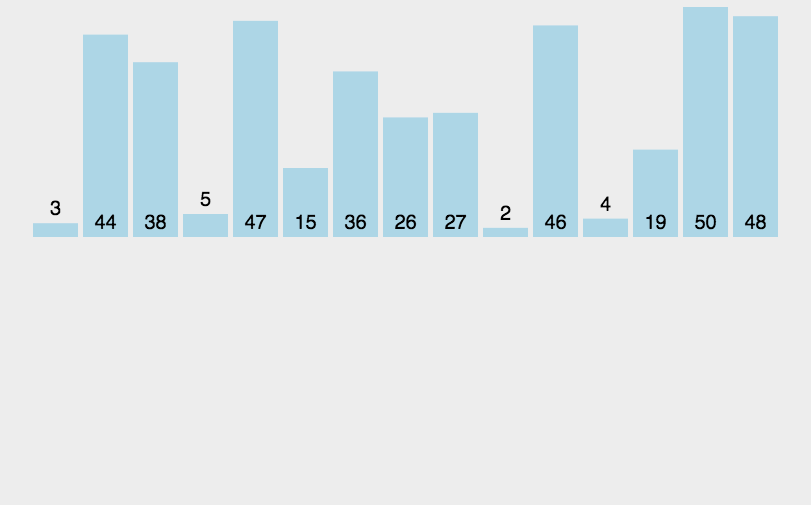①. 申请空间，使其大小为两个已经排序序列之和，该空间用来存放合并后的序列
②. 设定两个指针，最初位置分别为两个已经排序序列的起始位置
③. 比较两个指针所指向的元素，选择相对小的元素放入到合并空间，并移动指针到下一位置
④. 重复步骤③直到某一指针到达序列尾
⑤. 将另一序列剩下的所有元素直接复制到合并序列尾

3、代码实现

• 分解：将序列每次折半拆分
• 合并：将划分后的序列段两两排序合并

L[first…mid]为第一段，L[mid+1…last]为第二段，并且两端已经有序，现在我们要将两端合成达到L[first…last]并且也有序。

O(nlog₂n) O(nlog₂n) O(nlog₂n) O(n)

1、基本思想

• MSD（Most significant digital） 从最左侧高位开始进行排序。先按k1排序分组, 同一组中记录, 关键码k1相等, 再对各组按k2排序分成子组, 之后, 对后面的关键码继续这样的排序分组, 直到按最次位关键码kd对各子组排序后. 再将各组连接起来, 便得到一个有序序列。MSD方式适用于位数多的序列

• LSD （Least significant digital）从最右侧低位开始进行排序。先从kd开始排序，再对kd-1进行排序，依次重复，直到对k1排序后便得到一个有序序列。LSD方式适用于位数少的序列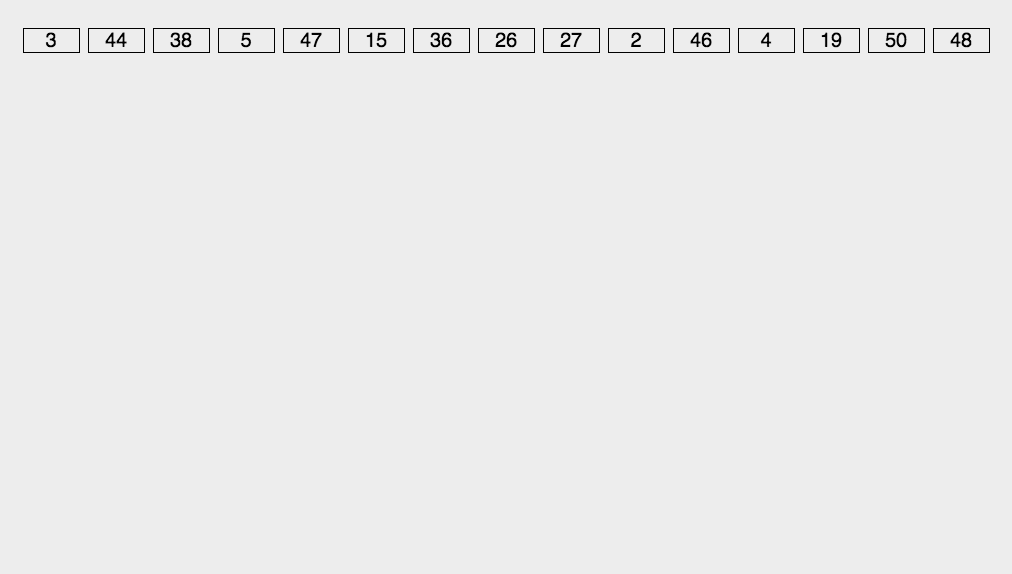2、算法描述

①. 取得数组中的最大数，并取得位数；

3、代码实现

• 分配：我们将L[i]中的元素取出，首先确定其个位上的数字，根据该数字分配到与之序号相同的桶中

• 收集：当序列中所有的元素都分配到对应的桶中，再按照顺序依次将桶中的元素收集形成新的一个待排序列L[]。对新形成的序列L[]重复执行分配和收集元素中的十位、百位…直到分配完该序列中的最高位，则排序结束

O(d*(n+r)) O(d*(n+r)) O(d*(n+r)) O(n+r)

Tips: 基数排序不改变相同元素之间的相对顺序，因此它是稳定的排序算法。

1. 基数排序：根据键值的每位数字来分配桶
2. 计数排序：每个桶只存储单一键值
3. 桶排序：每个桶存储一定范围的数值

总结

(1). 平方阶O(n²)排序：各类简单排序：直接插入、直接选择和冒泡排序

(2). 线性对数阶O(nlog₂n)排序：快速排序、堆排序和归并排序

(3). O(n1+§))排序，§是介于0和1之间的常数：希尔排序

(4). 线性阶O(n)排序：基数排序，此外还有桶、箱排序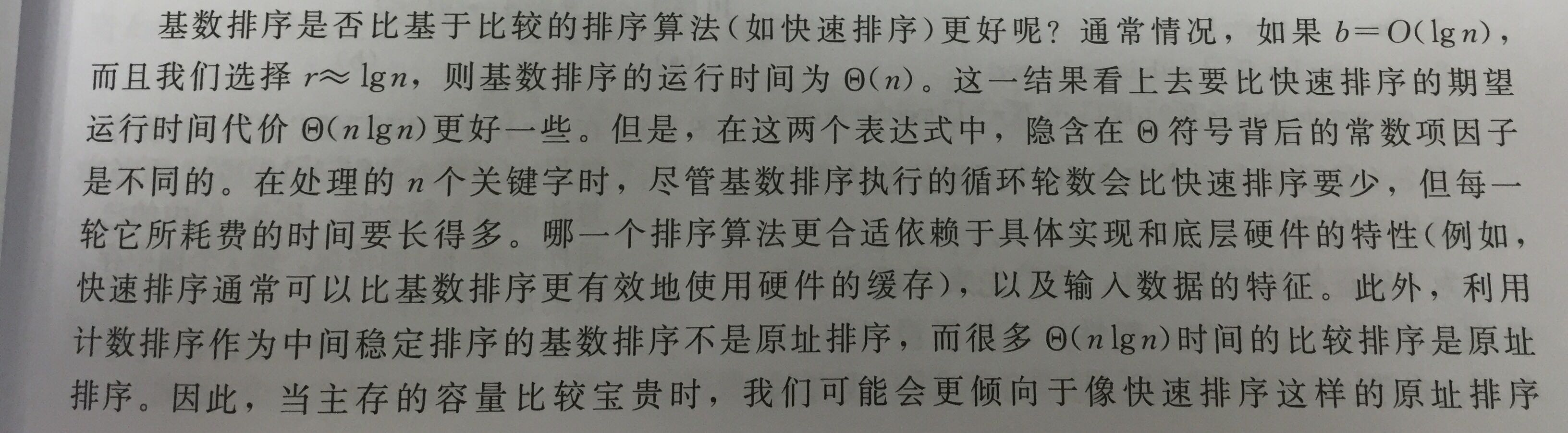时间复杂度极限

1. 计数排序 复杂度O( k+n) 要求：被排序的数是0~k范围内的整数
2. 基数排序 复杂度O( d(k+n) ) 要求：d位数，每个数位有k个取值
3. 桶排序 复杂度 O( n ) （平均） 要求：被排序数在某个范围内，并且服从均匀分布

说明

• 当原表有序或基本有序时，直接插入排序和冒泡排序将大大减少比较次数和移动记录的次数，时间复杂度可降至O（n）；
• 而快速排序则相反，当原表基本有序时，将蜕化为冒泡排序，时间复杂度提高为O（n2）；
• 原表是否有序，对简单选择排序、堆排序、归并排序和基数排序的时间复杂度影响不大。